# 7.6: Graphing Equations in Slope-Intercept Form

$$\newcommand{\vecs}{\overset { \rightharpoonup} {\mathbf{#1}} }$$ $$\newcommand{\vecd}{\overset{-\!-\!\rightharpoonup}{\vphantom{a}\smash {#1}}}$$$$\newcommand{\id}{\mathrm{id}}$$ $$\newcommand{\Span}{\mathrm{span}}$$ $$\newcommand{\kernel}{\mathrm{null}\,}$$ $$\newcommand{\range}{\mathrm{range}\,}$$ $$\newcommand{\RealPart}{\mathrm{Re}}$$ $$\newcommand{\ImaginaryPart}{\mathrm{Im}}$$ $$\newcommand{\Argument}{\mathrm{Arg}}$$ $$\newcommand{\norm}{\| #1 \|}$$ $$\newcommand{\inner}{\langle #1, #2 \rangle}$$ $$\newcommand{\Span}{\mathrm{span}}$$ $$\newcommand{\id}{\mathrm{id}}$$ $$\newcommand{\Span}{\mathrm{span}}$$ $$\newcommand{\kernel}{\mathrm{null}\,}$$ $$\newcommand{\range}{\mathrm{range}\,}$$ $$\newcommand{\RealPart}{\mathrm{Re}}$$ $$\newcommand{\ImaginaryPart}{\mathrm{Im}}$$ $$\newcommand{\Argument}{\mathrm{Arg}}$$ $$\newcommand{\norm}{\| #1 \|}$$ $$\newcommand{\inner}{\langle #1, #2 \rangle}$$ $$\newcommand{\Span}{\mathrm{span}}$$$$\newcommand{\AA}{\unicode[.8,0]{x212B}}$$

## Using the Slope and Intercept to Graph a Line

When a linear equation is given in the general form, $$ax+by=c$$, we observed that an efficient graphical approach was the intercept method. We let $$x=0$$ and computed the corresponding value of $$y$$, then let $$y=0$$ and computed the corresponding value of $$x$$.

When an equation is written in the slope-intercept form, $$y=mx+b$$, there are also efficient ways of constructing the graph. One way, but less efficient, is to choose two or three $$x$$-values and compute to find the corresponding $$y$$-values. However, computations are tedious, time consuming, and can lead to errors. Another way, the method listed below, makes use of the slope and the $$y$$-intercept for graphing the line. It is quick, simple, and involves no computations.

##### Graphing Method
1. Plot the $$y$$-intercept $$(0, b)$$.
2. Determine another point by using the slope m.
3. Draw a line through the two points.

Recall that we defined the slope $$m$$ as the ratio $$\dfrac{y_2-y_1}{x_2-x_1}$$. The numerator $$y_2−y_1$$ represents the number of units that $$y$$ changes and the denominator $$x_2 - x_1$$ represents the number of units that $$x$$ changes. Suppose $$m=pq$$. Then $$p$$ is the number of units that $$y$$ changes and $$q$$ is the number of units that $$x$$ changes. Since these changes occur simultaneously, start with your pencil at the $$y$$-intercept, move $$p$$ units in the appropriate vertical direction, and then move $$q$$ units in the appropriate horizontal direction. Mark a point at this location.

## Sample Set A

Graph the following lines.

##### Example $$\PageIndex{1}$$

$$y = \dfrac{3}{4}x + 2$$

1. The $$y$$-intercept is the point $$(0,2)$$. Thus the line crosses the $$y$$-axis $$2$$ units above the origin. Mark a point at $$(0,2)$$.2. The slope, $$m$$, is $$\dfrac{3}{4}$$. This means that if we start at any point on the line and move our pencil $$3$$ units up and then $$4$$ units to the right, we’ll be back on the line. Start at a known point, the $$y$$-intercept $$(0, 2)$$. Move up $$3$$ units, then move $$4$$ units to the right. Mark a point at this location. (Note also that \dfrac{3}{4} = \dfrac{-3}{-4}\). This means that if we start at any point on the line and move our pencil $$3$$ units down and $$4$$ units to the left, we’ll be back on the line. Note also that $$\dfrac{3}{4} = \dfrac{\dfrac{3}{4}}{1}$$. This means that if we start at any point on the line and move to the right $$1$$ unit, we’ll have to move up $$\dfrac{3}{4}$$ unit to get back on the line.)3. Draw a line through both points.##### Example $$\PageIndex{2}$$

$$y = -\dfrac{1}{2}x + \dfrac{7}{2}$$

1. The $$y$$-intercept is the point $$(0, \dfrac{7}{2})$$. Thus the line crosses the $$y$$-axis $$\dfrac{7}{2}$$ units above the origin. Mark a point $$(0, \dfrac{7}{2})$$, or $$(0, 3\dfrac{1}{2})$$.2. The slope, $$m$$, is $$-\dfrac{1}{2}$$. We can write $$-\dfrac{1}{2}$$ as $$\dfrac{-1}{2}$$. Thus, we start at a known point, the $$y$$-intercept $$(0, 3\dfrac{1}{2})$$, move down one unit (because of the $$-1$$), then move right $$2$$ units. Mark a point at this location.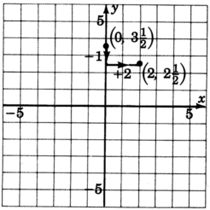3. Draw a line through both points.##### Example $$\PageIndex{3}$$

$$y = \dfrac{2}{5}x$$

1. We can put this equation into explicit slope-intercept by writing it as $$y = \dfrac{2}{5}x + 0$$.

The $$y$$-intercept is at the point $$(0, 0)$$, the origin. This line goes right through the origin.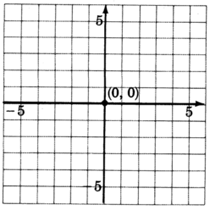2. The slope, $$m$$, is $$\dfrac{2}{5}$$. Starting at the origin, we move up $$2$$ units, then move to the right $$5$$ units. Mark a point at this location.3. Draw a line through the two points.

##### Example $$\PageIndex{4}$$

$$y = 2x - 4$$

1. The $$y$$-intercept is the point $$(0, -4)$$. Thus the line crosses the $$y$$-axis $$4$$ units below the origin. Mark a point at $$(0, -4)$$.2. The slope, $$m$$, is $$2$$. If we write the slope as a fraction, $$2 = \dfrac{2}{1}$$, we can read how to make the changes. Start at the known point $$(0, -4)$$, move up $$2$$ units, then move right $$1$$ unit. Mark a point at this location.3. Draw a line through the two points.

## Practice Set A

Use the $$y$$-intercept and the slope to graph each line.

##### Practice Problem $$\PageIndex{1}$$

$$y = \dfrac{-2}{3} + 4$$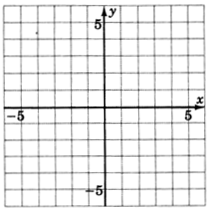##### Practice Problem $$\PageIndex{2}$$

$$y = \dfrac{3}{4}x$$## Exercises

For the following problems, graph the equations.

##### Exercise $$\PageIndex{1}$$

$$y = \dfrac{2}{3} + 1$$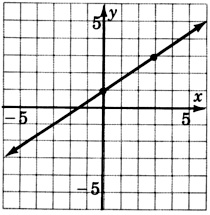##### Exercise $$\PageIndex{2}$$

$$y = \dfrac{1}{4}x - 2$$##### Exercise $$\PageIndex{3}$$

$$y = 5x - 4$$##### Exercise $$\PageIndex{4}$$

$$y = -\dfrac{6}{5} - 3$$##### Exercise $$\PageIndex{5}$$

$$y = \dfrac{3}{2} - 5$$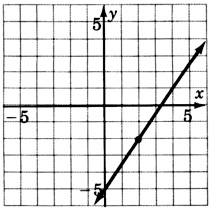##### Exercise $$\PageIndex{6}$$

$$y = \dfrac{1}{5}x + 2$$##### Exercise $$\PageIndex{7}$$

$$y = -\dfrac{8}{3} + 4$$##### Exercise $$\PageIndex{8}$$

$$y = -\dfrac{10}{3} + 6$$##### Exercise $$\PageIndex{9}$$

$$y = 1x - 4$$##### Exercise $$\PageIndex{10}$$

$$y = -2x + 1$$##### Exercise $$\PageIndex{11}$$

$$y = x + 2$$##### Exercise $$\PageIndex{12}$$

$$y = \dfrac{3}{5}x$$##### Exercise $$\PageIndex{13}$$

$$y = -\dfrac{4}{3}$$##### Exercise $$\PageIndex{14}$$

$$y = x$$##### Exercise $$\PageIndex{15}$$

$$y = -x$$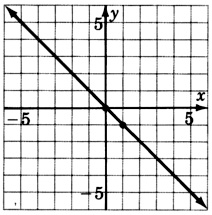##### Exercise $$\PageIndex{16}$$

$$3y−2x=−3$$##### Exercise $$\PageIndex{17}$$

$$6x+10y=30$$##### Exercise $$\PageIndex{18}$$

$$x+y=0$$## Exercises for Review

##### Exercise $$\PageIndex{19}$$

Solve the inequality $$2 - 4x \ge x - 3$$

$$x≤1$$

##### Exercise $$\PageIndex{20}$$

Graph the inequality $$y+3>1.$$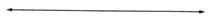##### Exercise $$\PageIndex{21}$$

Graph the equation $$y = -2$$.##### Exercise $$\PageIndex{22}$$

Determine the slope and $$y$$-intercept of the line $$−4y−3x=16$$.

##### Exercise $$\PageIndex{23}$$

Find the slope of the line passing through the points $$(−1, 5)$$ and $$(2, 3)$$.

$$m = \dfrac{-2}{3}$$